Start typing, then use the up and down arrows to select an option from the list.# Calculate Oxidation Numbers Example 4

Jules Bruno
205views
2
here, It says, give the oxidation number of the carbon atoms in the acetate ion. All right, so we're gonna say we're looking for carbon, so carbon is going to be our X hydrogen, when it's with non metals is plus one and then oxygen here in It's not an ox, super oxide or peroxide. So it's minus two. So we have two carbons. Each one is X plus three hydrogen. Each one is plus one plus two oxygen's. Each one is minus two. This equation equals the charge of the ion, which is minus one. So all we have to do now solve for X so two X plus three minus four equals negative one two X minus one equals negative one. So add one to both sides here. So here, you know, weird turn of events. You can see that X now equals zero. So here the oxidation number off carbon within the structure is zero. So there are moments where you're oxidation number could be zero, and the element is not not not in its natural state, such as in this example. So again, the oxidation number of carbon when we calculated it comes out to be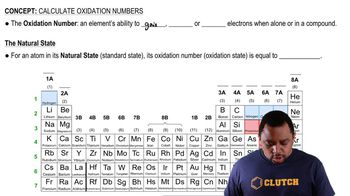02:42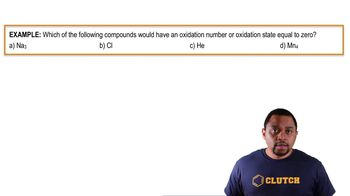01:1601:1501:38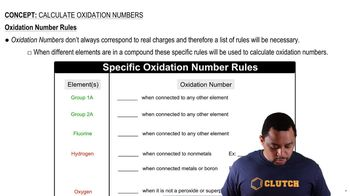03:13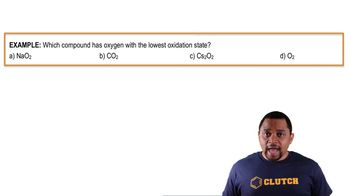01:3601:09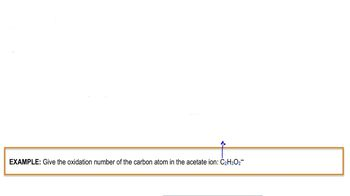01:22